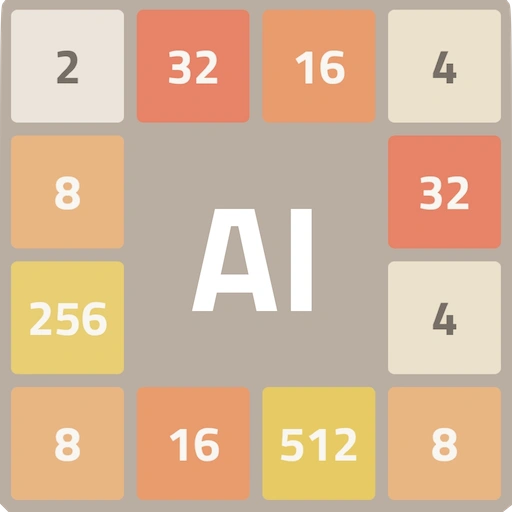## 2048 Game

2048 is a game where you combine tiles to get a tile with the number 2048. The game is very simple, but it is surprisingly addictive and challenging.

There are many different AI algorithms that can be used to play 2048.

In this post, we will go through the different algorithms, find out which algorithm is the best for 2048, and how to tune the parameters to get the best results.

You can play the game AI Simulator: 2048 to try out the different algorithms described in this post.

## Basic Heuristic Algorithm

Basic Heuristic (HEUR) algorithm is a simple algorithm that uses a few heuristics to decide the best move. It is a good starting point for beginners to understand how the parameters affect the outcome of the AI decisions.

The best settings for HEUR algorithm involves using the following parameters:

• Delta factor: Directly consider the score of the move. Higher delta factor means the AI will prefer moves that increase the score. This is usually a good setting to have above 1.
• Smooth factor: Consider the smoothness of the board, i.e. number of adjacent cells that can be merged together. Higher smooth factor means the AI will prefer moves that make the board smoother and easier to merge. This is also a good setting to have above 1.

Other parameters such as empty cells factor can be occasionally useful, but they are not as important as the two parameters above.

Overall, Basic Heuristic algorithm is a good starting point for beginners, but it is not very powerful.

Advanced Heuristic algorithms such as Expectimax (EXPM) are more powerful than the Basic Heuristic algorithm, as they take into account multiple moves ahead, instead of just a single move. This allows the AI to make better decisions and avoid bad moves.

However, the downside is that the AI will take longer to decide on a move, as it needs to simulate many possible moves to decide the best move.

The best settings for EXPM algorithm involves using the following parameters:

• Maximum depth: The maximum number of moves the AI will simulate. Higher maximum depth means the AI will take longer to decide on a move, but it will be able to make better decisions. While having a large maximum depth is good, it is also important to have a good time limit, so that the AI does not take too long to decide on a move. The default maximum depth is 3, which is a good starting point. You can increase the maximum depth to 4 or 5 if your device is powerful enough.

Advanced Heuristic algorithms are more powerful than Basic Heuristic algorithms, but they too are limited by the heuristics being used and the number of moves being simulated.

## Monte Carlo Methods

Monte Carlo Methods such as Monte Carlo Tree Search (MCTS) and Pure Monte Carlo Game Search (PMGS) are more powerful than the Advanced Heuristic algorithms, as they simulate many possible moves and choose the best move based on the results of the simulations, without relying on heuristics.

However, the downside is that the AI will take even longer to decide on a move, as it needs to simulate even more moves to decide the best move.

The name “Monte Carlo” has an interesting origin. According to Wikipedia, Monte Carlo Method was named after the Monte Carlo Casino in Monaco.

The best settings for PMGS algorithm involves using the following parameters:

• Number of games to simulate: The number of games the AI will simulate. Higher number of games means the AI will take longer to decide on a move, but it will be able to make better decisions. The default value is 5, but you can increase it to 20 or 50 if your device is powerful enough.

Monte Carlo Methods are more powerful than Advanced Heuristic algorithms, and they are the best class of algorithms to use for 2048 in the AI Simulator: 2048 game.

## Machine Learning

Machine Learning algorithms such as Deep Q-Learning (DQN) and Proximal Policy Optimization (PPO) are examples of reinforcement learning algorithms that use neural networks to learn how to play the game. They learn by playing the game many times, and improving their decisions based on the results of the games (reward function).

While theoretically machine Learning algorithms are the most powerful, DQN algorithm is shown to be less powerful than the Monte Carlo Methods in practice for the 2048 game.

There could be several reasons for this:

• It takes a long time to train the model. So while it is possible that a well-trained neural network can play the game at a very high level, it might take weeks or months to train the neural network.
• The game mechanics of 2048 is simple enough that it is “solvable” by using non-machine learning algorithms such as Monte Carlo Methods.

The best parameters and settings for DQN algorithm is covered in-depth in the DQN page.

## 2048 Best Algorithm

In summary, the best algorithm for 2048 is Monte Carlo Methods, specifically PMGS algorithm.

## Credits